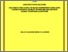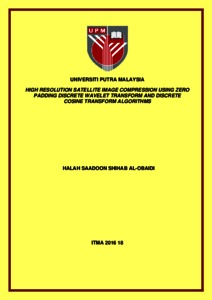# High resolution satellite image compression using zero padding discrete wavelet transform and discrete cosine transform algorithms

## Citation

Al-Obaidi, Halah Saadoon Shihab (2016) High resolution satellite image compression using zero padding discrete wavelet transform and discrete cosine transform algorithms. Masters thesis, Universiti Putra Malaysia.

## Abstract

Raw satellite images are considered high in resolution, especially multispectral images captured by remote sensing satellites. Hence, choosing the suitable compression technique for such images should be carefully considered, to achieve high values of compression ratio (CR) to decrease the data storage on-board satellites, and the bandwidth required to transmit data from the satellite to earth, while simultaneously maintaining the important scientific information of the image when reconstructed at the ground station. The Discrete Cosine Transform (DCT) and the Discrete Wavelet Transform (DWT)-based compression techniques have been utilized in most of the space missions launched throughout the last few decades due to their efficiency. However, both techniques have some drawbacks that should be addressed, such as blocking artefacts for DCT and computational complexity for DWT. Several different hybrid compression methods based on DCT and DWT were used in previous works to utilize the positive properties and decrease the effect of the negative properties of these transforms. However, none of these hybrid methods were implemented on high resolution satellite images, and each method had one or more shortcomings. Hence, a proposed hybrid (DWT-DCT) method was presented and implemented in the current work on several satellite images, simulating an image compression system on-board a small satellite. This method can achieve higher values of compression ratio, through adding the 2D-DCT to the 2D-DWT second level coefficients instead of further complex levels of 2D-DWT compression. Besides, this method can maintain an acceptable reconstructed image quality through replacing the standard DWT thresholding and quantization processes with an alternative process that employed the zero-padding technique in a way that controlled the change in Compression Ratio (CR) and Peak Signal to Noise Ratio (PSNR), and helped to decrease the DWT processing time. Image compression was performed on several satellite images using DCT, DWT, and the proposed Hybrid method with the aim of comparing the results. Hence, it was indicated that the proposed hybrid (DWT-DCT) technique achieved better performance than the standalone DCT or DWT techniques. All the results were obtained objectively (by calculating the CR and PSNR values for each case using the MATLAB software package), and subjectively (Visually). A gain of 43.6%, 57.8% and 96.5% of CR was achieved using the proposed method relative to the DWT, for the satellite images Baghdad, Basra and Erbil respectively, with a negligible reduction in the reconstructed image quality. Hence, it was concluded that using the proposed method improved the satellite image compression, and it is feasible to be used onboard satellites.Preview
Text
ITMA 2016 18 IR.pdfView Item